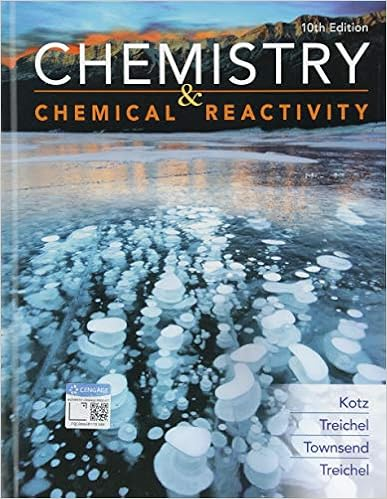# Predict which solute is more soluble in the given

• Test Prep
• 7
• 100% (2) 2 out of 2 people found this document helpful

This preview shows page 6 - 7 out of 7 pages.

##### We have textbook solutions for you!
The document you are viewing contains questions related to this textbook.The document you are viewing contains questions related to this textbook.
Chapter 22 / Exercise 25
Chemistry & Chemical Reactivity
Kotz/TreichelExpert Verified
51. Predict which solute is more soluble in the given solvent. (I) 1,4-butanediol (HOCH2CH2CH2CH2OH) or butanol (CH3CH2CH2CH2OH) in water (II) Chloroform (CHCl3) or carbon tetrachloride (CCl4) in water I II produced by the reaction of 60.0 g of aluminum oxide with enough amount of carbon according to the following chemical reaction? Al2O3+ 3C 2Al + 3CO 56. The combustion of propane, C3H8, produces CO2and H2O: C3H8(g) + 5O2(g) 3CO2(g) + 4H2O (g) A. 1,4-butanediol chloroform B. 1,4-butanediol carbon tetrachlorideC. butanol chloroform D. butanol carbon tetrachloride
52. Balance the following equation using the smallest set of whole numbers. What is the balancing coefficient of H2O? __KMnO4+ __HCl __KCl + __MnCl4+ __H2O + __Cl22O will be produced if 2.5 moles of O2and an excess of C3H8are used in the reaction? 57. How many sulfur atoms are in 25.6 g of Al2(S2O3)3? 58. How many grams of aluminum are in 25.6 g of Al2(S2O3)3? 59. 7.53 × 1023molecules of an element has a mass of 88.64 g. What is the identity of this molecular element? 53. What is the sum of the coefficients when the following reaction is balanced using the simplest whole number coefficients?
54. A mixture of two elements and their reaction product is represented below. For a different experiment, how many moles of the product can be produced if 3.0 moles of are reacted with excess ? Consider writing a balanced equation first.
55. What is the mass of aluminum that can be produced by the reaction of 60.0 g of aluminum oxide with enough amount of carbon according to the following chemical reaction? Al2O3+ 3C 2Al + 3CO 56. The combustion of propane, C3H8, produces CO2and H2O: C3H8(g) + 5O2(g) 3CO2(g) + 4H2O (g) How many moles of H2O will be produced if 2.5 moles of O2and an excess of C3H8are used in the reaction? 57. How many sulfur atoms are in 25.6 g of Al2(S2O3)3? 58. How many grams of aluminum are in 25.6 g of Al2(S2O3)3? 59. 7.53 × 1023molecules of an element has a mass of 88.64 g. What is the identity of this molecular element?
##### We have textbook solutions for you!
The document you are viewing contains questions related to this textbook.The document you are viewing contains questions related to this textbook.
Chapter 22 / Exercise 25
Chemistry & Chemical Reactivity
Kotz/TreichelExpert Verified
CH 101 Mock Exam 2 USEFUL INFORMATION: NA= 6.0221 x 10231 amu = 1.66054 × 1024g _______________________________________________________________________________________ Electron Geometries and Molecular Shapes from VSEPR (Valence Shell Electron Pair Repulsion Model) # electron domains (steric number) Electron Geometry (structural-pair) geometry Molecular Shape 5 trigonal bipyramidal trigonal bipyramidal 5 trigonal bipyramidal see-saw 5 trigonal bipyramidal T-shaped 5 trigonal bipyramidal linear 6 octahedral octahedral 6 octahedral square pyramidal 6 octahedral square planar
•••